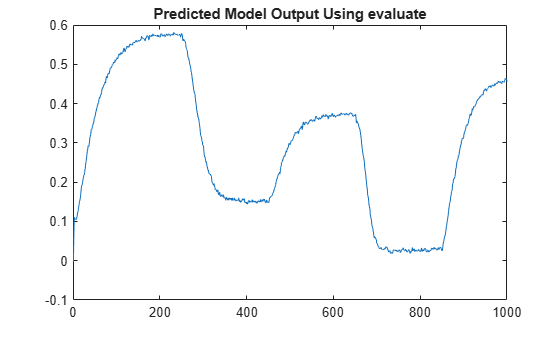# getreg

Regressor expressions and numerical values in nonlinear ARX model

## Syntax

```Rs = getreg(model) Rm = getreg(model,data) Rm = getreg(model,data,init) Rm = getreg(___,'Type',regressorType) ```

## Description

`Rs = getreg(model)` returns expressions for computing regressors in the nonlinear ARX model. `model` is an `idnlarx` object. A typical use of the regression matrices built by `getreg` is to generate input data when you want to evaluate the output of a mapping function such as `idWaveletNetwork` using `evaluate`. For example, the following pair of commands evaluates the output of a mapping function `model`.

```Regressor_Value = getreg(model,data,'z') y = evaluate(model.OutputFcn,RegressorValue)```
These commands are equivalent to the command:
`y = predict(model,data,1,predictOptions('InitialCondition','z'))`

`Rm = getreg(model,data)` returns regressor values as a `timetable` for the specified input/output data set `data`.

`Rm = getreg(model,data,init)` uses the initial conditions that are specified in `init`. The first `N` rows of each regressor matrix depend on the initial states `init`, where `N` is the maximum delay in the regressors (see `getDelayInfo`).

`Rm = getreg(___,'Type',regressorType)` returns the names of the regressors of the specified `regressorType`. For example, use the command `Rm = getreg(model,'Type','input')` to return the names of only the input regressors.

## Input Arguments

`data`

`iddata` object containing measured data or numeric matrix that contains the values of the output and input variables in the order ```[model.OutputName model.InputName]```.

`init`

• `'z'` (default) specifies zero initial state.

• `NaN` denotes unknown initial conditions.

• Real column vector containing the initial state values. For more information on initial states, see Definition of idnlarx States in `idnlarx`. For multiple-experiment data, this is a matrix where each column specifies the initial state of the model corresponding to that experiment.

• `iddata` object containing input and output samples at time instants before to the first sample in `data`. When the `iddata` object contains more samples than the maximum delay in the model, only the most recent samples are used. The number of samples required is equal to `max(getDelayInfo(model))`.

`model`

`iddata` object representing nonlinear ARX model.

`regressorType`

Type of regressor to return, specified as one of the following:

• `'all'` (default) — All regressors

• `'input'` — Only input regressors

• `'output'` — Only output regressors

• `'standard'` — Only linear and polynomial regressors

• `'custom'` — Only custom regressors

## Output Arguments

`Rm`

`timetable` of regressor values for all or a specified subset of regressors. Each column in `Rm` contains as many rows as there are data samples. For a model with `nr` regressors, `Rm` contains one column for each regressor. When `data` contains multiple experiments, `Rm` is a cell array where each element corresponds to a timetable of regressor values for an experiment.

`Rs`

Regressor expressions represented as a cell array of character vectors. For example, the expression `'u1(t-2)'` computes the regressor by delaying the input signal `u1` by two time samples. Similarly, the expression `'y2(t-1)'` computes the regressor by delaying the output signal `y2` by one time sample.

The order of regressors in `Rs` corresponds to regressor indices in the `idnlarx` object property `model.RegressorUsage`.

## Examples

collapse all

Load sample data u and y.

``` load twotankdata; Ts = 0.2;```

Sample time is 0.2 sec.

Create data object and use first 1000 samples for estimation.

``` z = iddata(y,u,Ts); ze = z(1:1000);```

Estimate nonlinear `ARX` model.

` model = nlarx(ze,[3 2 1]);`

Get regressor expressions.

` Rs = getreg(model)`
```Rs = 5x1 cell {'y1(t-1)'} {'y1(t-2)'} {'y1(t-3)'} {'u1(t-1)'} {'u1(t-2)'} ```

Get regressor values.

` Rm = getreg(model,ze)`
```Rm=1000×5 timetable Time y1(t-1) y1(t-2) y1(t-3) u1(t-1) u1(t-2) _______ ________ ________ ________ _______ _______ 0.2 sec 0 0 0 0 0 0.4 sec 0.1003 0 0 10 0 0.6 sec 0.094621 0.1003 0 10 10 0.8 sec 0.084424 0.094621 0.1003 10 10 1 sec 0.081449 0.084424 0.094621 10 10 1.2 sec 0.08546 0.081449 0.084424 10 10 1.4 sec 0.083002 0.08546 0.081449 10 10 1.6 sec 0.08443 0.083002 0.08546 10 10 1.8 sec 0.092793 0.08443 0.083002 10 10 2 sec 0.099804 0.092793 0.08443 10 10 2.2 sec 0.10559 0.099804 0.092793 10 10 2.4 sec 0.1081 0.10559 0.099804 10 10 2.6 sec 0.12108 0.1081 0.10559 10 10 2.8 sec 0.12404 0.12108 0.1081 10 10 3 sec 0.13551 0.12404 0.12108 10 10 3.2 sec 0.13405 0.13551 0.12404 10 10 ⋮ ```

Evaluate and plot model output for one-step-prediction.

``` Y = evaluate(model.OutputFcn,Rm.Variables); plot(1:1000,Y) title('Predicted Model Output Using evaluate')```The previous result is equivalent to the result obtained by using `predict` in the following commands.

``` Y_p = predict(model,ze,1,'z'); Y = Y_p.OutputData; plot(Y) title('Predicted Model Output Using predict')```## Version History

Introduced in R2007a

expand all

Behavior changed in R2021a